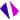# 導數章節介紹課程內容
3.1 derivative
3.2 differentiation formulas
3.3 the chain rule
3.4 Derivatives of implicit and inverse functions
3.5 derivative of exponential function and logarithmic functions
3.6 derivatives of trigonometric and hyperbolic functions
3.7 higher-order derivatives
 課程講義 課程影音3.1 節講義3.2 節講義3.3 節講義3.4 節講義3.5 節講義3.6 節講義3.7 節講義3.1 derivative3.2 differentiation formulas_013.2 differentiation formulas_023.3 the chain rule3.4 Derivatives of implicit and inverse functions3.7 higher-order derivatives